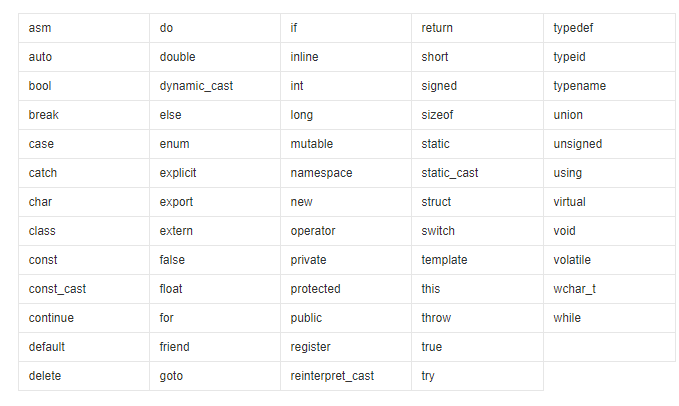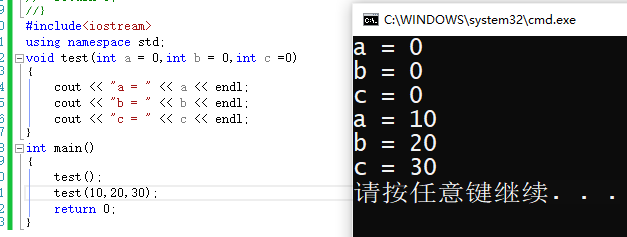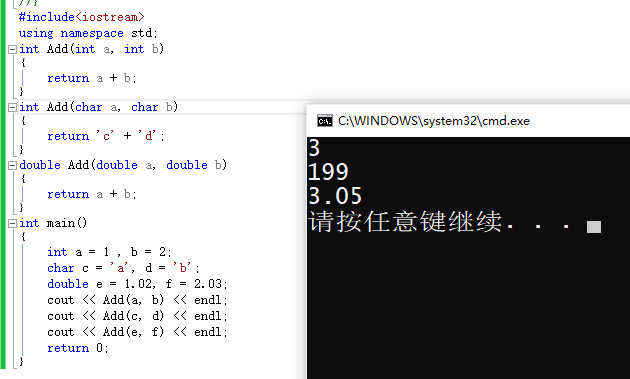# C++入门，初篇

2021/7/20 15:25:44 浏览：

### 文章目录

• 前言
• 一、C++关键字
• 二、命名空间
• 1.std空间
• 2.其他自己定义的空间
• 三、C++输入输出
• 四、C++中的缺省参数
• 1.C++缺省参数的意义
• 2.C++缺省参数的分类
• 五、C++中的函数重载
• 1.函数重载的定义
• 2.函数重载的实现
• 六、C++中的引用
• 1.引用的实现
• 2.引用和指针的比较
• 六、内联函数
• 总结

# 一、C++关键字

1.C++发展至今，已经有C++98，C++11和C++17这几个主流版本。在C++98中总共有63个关键字，其中有32个是从C语言中继承过来的。# 二、命名空间

## 1.std空间

namespace std库标准命名空间，包括C++标准库中的所有表示符

``````#include<iostream>
using namespace std;
int main()
{
cout << "hello world" << endl;
}
//比如里面用到的cout和endl就属于std库中，你如果不声明使用std库的话，就需要用
//作用域限定符(::)声明引入。
#include<iostream>
int main()
{
std::cout << "hello world" << std::endl;
}
``````

## 2.其他自己定义的空间

``````#include<iostream>
namespace myspace
{
int a = 100;
int b = 300;
{
return a + b;
}
void print()
{
}
}
using namespace myspace;
int main()
{
myspace::print(); //用作用域限定符说明调用的是myspace空间里的print函数
print(); //直接用using namespace关键字引入
return 0;
}
``````

# 三、C++输入输出

C有自己的输入和输出，C++也有自己的输入输出。

``````#include<stdio.h>
int main()
{
printf("hello world\n");
return 0;
}
#include<stdio.h>
int main()
{
char s;
scanf("%s", s);
printf("%s\n",s);
return 0;
}
``````

C语言对于输出输入有较高的格式输出要求，就是你在输出时必须声明他是以何种格式输出的，否则他就会报错或者认为你需要输入的就是你屏幕上的一个字符串。
C++在对C的输入输出继承的情况下，重写了输入输出，对于输出输入格式没有要求，在输入自定义类型时，需要你将输入和输出运算符重载。

``````#include<iostream>
using namespace std;
int main()
{
int a;
float b;
cin >> a >> b;
cout << "a = " << a << endl;
cout << "b = " << b << endl;
}
``````# 四、C++中的缺省参数

## 1.C++缺省参数的意义

``````#include<iostream>
using namespace std;
void test(int a = 0)
{
cout << "a = " << a << endl;
}
int main()
{
test();//在不传时，他就会使用缺省参数
test(10);//传参时，使用时指定的实参
return 0;
}
``````

## 2.C++缺省参数的分类

``````#include<iostream>
using namespace std;
void test(int a = 0,int b = 0,int c =0)
{
cout << "a = " << a << endl;
cout << "b = " << b << endl;
cout << "c = " << c << endl;
}
int main()
{
test();
test(10,20,30);
return 0;
}
````````````#include<iostream>
using namespace std;
void test(int a ,int b = 0,int c =0)
{
cout << "a = " << a << endl;
cout << "b = " << b << endl;
cout << "c = " << c << endl;
}
int main()
{
test(1);//你对b和c都给了缺省参数，但是a没有缺省参数，你需要传入a的值
test(10,20,30);//你传入三个值，则不使用你给定的缺省参数。
return 0;
}
``````

# 五、C++中的函数重载

## 1.函数重载的定义# 六、C++中的引用

## 1.引用的实现

1.引用在定义时必须初始化，
2.一个变量可以有多个引用，但是一个引用只能引用一个实体，且是他的整个生命周期。

``````#include<iostream>
using namespace std;
int main()
{
int a = 10;
int& b = a; //b的存在时间是整个函数，且只能是实体a的引用
cout << b << endl;
}
``````

## 2.引用和指针的比较

``````#include<iostream>
using namespace std;
int main()
{
int a = 10;
int& b = a;
int* c;
c = &a;
cout << b << endl;
cout <<*c << endl;
return 0;
}
``````

1.引用在定义的时候就需要初始化，指针的话没有要求必须初始化，就是你可以指向空，也可以不给，也可以指向一个对象。
2.引用在初始化之后，他就不能再去引用别的对象了，指针的话可以指向同种类型的别的对象。
3.存在空指针，但是不存在空引用
4.在测量长度的时候，引用的大小是其指向实体的大小，而指针的大小永远是寻址范围的大小，（32位下为4字节长度，64位下为8字节长度）。
5.存在多级的指针，但是只存在一级的引用。
6.访问实体空间，引用的话直接是编译器处理，指针需要你对其进行解引用。
7.引用比指针安全，指针存在野指针，但是引用的话，不存在野引用。